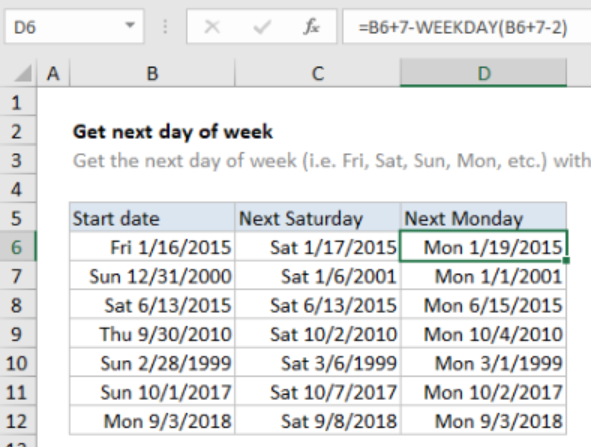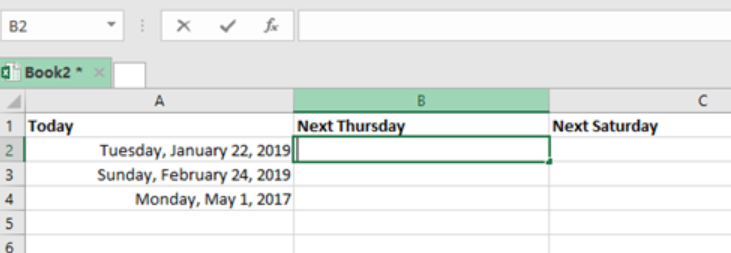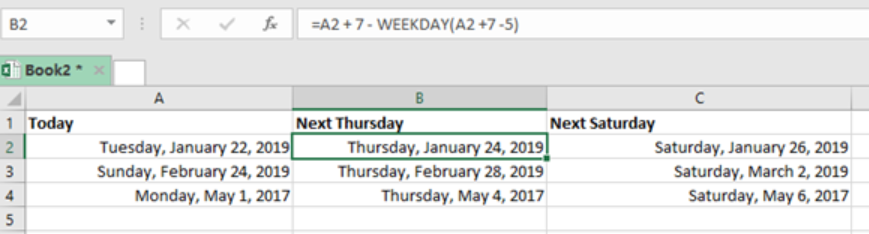Get instant live expert help with Excel or Google Sheets“My Excelchat expert helped me in less than 20 minutes, saving me what would have been 5 hours of work!”

#### Post your problem and you’ll get expert help in seconds.

Your message must be at least 40 characters
Our professional experts are available now. Your privacy is guaranteed.

# Get next day of week

We can use a formula that is based on the WEEKDAY function to get next day of week. All you will need is the start date, and then you will be able to effortlessly get the next day of the week. This article provides a clear guide on how we can get the next day of the week using a formula based on the WEEKDAY function.Figure 1: Getting next day of week

## General syntax of the formula

`=date + 7 – WEEKDAY(date + 7 –dow)`

Where;

• Date- this shows the date that excel is expected to start counting from. Here, we use a cell range.
• Dow- this is simply “day of week”, shortened as dow. This shows the day of the week that excel should count to.

## Understanding the formula

• In excel, each day has a specific number. It is from this fact that the concept of ‘weekday’ or ‘day of week’ is built.
• When working with excel in its default settings, you will notice that Sunday is set as 1, Monday is 2 all the way to Saturday as 7.
• For the function to return a number between 1 and 7, we must supply it with a valid date.

## How the formula works

• This formula is fundamental when it comes to finding the next day of the week.
• It does this by first rolling the date forward by 7 days
• Then, the function steps back to the correct date. This is done by subtracting the result of the calculation which uses the WEEKDAY function.

## Example

To find the next Thursday and Sunday in excel, we can proceed as follows;

Step 1: Enter the starting datesFigure 2: How to get next day of week using WEEKDAY function

Step 2: Use WEEKDAY function to get next day of weekFigure 3: Next days of week by WEEKDAY function

While working with the WEEKDAY function, remember to make the cells large enough to fit the dates data. This is because if the date data does not fit in the cells, then you will get a result that looks like this; ###########

You should also be keen to correctly enter the numerical value of the day, where

1- Sunday

2- Monday

3- Tuesday

4- Wednesday

5- Thursday

6- Friday

7- Saturday

## Instant Connection to an Expert through our Excelchat Service

Most of the time, the problem you will need to solve will be more complex than a simple application of a formula or function. If you want to save hours of research and frustration, try our live Excelchat service! Our Excel Experts are available 24/7 to answer any Excel question you may have. We guarantee a connection within 30 seconds and a customized solution within 20 minutes.

### Did this post not answer your question? Get a solution from connecting with the expert.Another blog reader asked this question today on Excelchat:
Solution examplesneed help with the different sheets and formula IF(A4=B4,0,NETWORKDAYS(A4+1,B4,\$E\$9:\$E\$42) +0.5*(INT((+B4-A4+1)/7)+IF(WEEKDAY(A4+1)+MOD (B4-A4-1,7)>=7,1,0)))
Solved by O. D. in 11 mins## Subscribe to Excelchat.coAnother blog reader asked this question today on Excelchat: